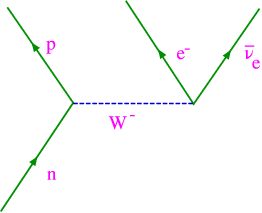### 7.3 Weak Force

This manifests itself through nuclear $\beta$ decay,

 $n\to p+{e}^{-}+\overline{{\nu }_{e}}.$ (7.7)

The standard coupling for this theory is called the Fermi coupling, ${G}_{F}$, after its discoverer. After the theory was introduced it was discovered that there were physical particles that mediate the weak force, the ${W}^{±}$ and the ${Z}^{0}$ bosons. These are very heavy particles (their mass is about 80 times the proton mass!), which is why they have such a small range – ﬂuctuations where I need to create that much mass are rare. The ${W}^{±}$ bosons are charged, and the ${Z}^{0}$ boson is neutral. The typical $\beta$ decay referred to above is mediated by a ${W}^{-}$ boson as can be seen in the Feynman diagram ﬁgure 7.1 . The reason for this choice is that it conserves charge at each point (the charge of a proton and a ${W}^{-}$ is zero, the charge of an electron and a neutrino is -1, the same as that of a ${W}^{-}$).Figure 7.1: The Feynman diagram for the weak decay of a neutron.